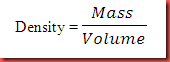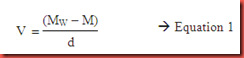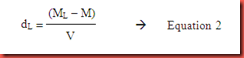## Saturday, April 28, 2012

### Determination of the Density of An Unknown Liquid

Objective:

1. To determine the density of a liquid using the pycnometer method

2. To identify the unknown liquid

Introduction:

Density is an elementary physical property of matter. It is defined as the ratio of mass to volume of a substance as shown in the following:Numerically it represents the mass per unit volume of matter. The SI unit of density is always expressed in the unit of kg m-3 or g cm-3. As the temperature increases, the density of a substance will be decreased, this will be discussed later in discussion. When thermal expansion occurs, the volume of the substance increases while its mass is remained. By referring to the formula given, the density of the substance decreases as the temperature increase. The density of a substance is varies with the change of temperature at surrounding.

In this experiment, the density of unknown liquid is determined by using pycnometer method. Density determination by pycnometer is a very precise method to determine the density of an unknown liquid. This experimental determination requires two important measurable quantities which are mass and volume of the selected piece of matter. The mass of the dry and empty pycnometer is represented by M and MW be the mass of the pycnometer filled with water at t°C. The mass of water in the pycnometer at this tempearature will be determined by using mass of pycnometer, MW minus mass of empty pycnometer, M. Let d be the density of water at t°C, so that the volume of pycnometer, V at this temperature can be expressed in the term as:The value of d can be refers to the Table 1 given.

Table 1 Density of pure water at different temperatures
 Temperature, °C Density, g cm-3 18 0.99862 20 0.99823 22 0.99780 24 0.99732

Let ML be the mass of pycnometer filled with the unknown liquid whose density is to be determined in this experiment. The mass of liquid is (ML – M). From equation 1, we can obtain the volume of empty pycnometer. The volume of pycnometer is equals to the volume of unknown liquid occupied. Thus, once we obtain the volume and mass of unknown liquid, the density of the unknown liquid, dL can be determined precisely. The density of unknown liquid, dL can be derived by substituted Equation 1 into Equation 2.Thus, the density of unknown liquid could be by using the formula as shown below:

A pycnometer (shown in Fig. 1) is a glass flask that has a close-fitting ground glass stopper with a capillary hole through it. This fine hole allows the release of a spare liquid after closing a top-filled pycnometer and the escape of air bubbles in the liquid. Besides, the fine hole allows for obtaining a given volume of measured and/or working liquid with a high accuracy.

Materials:

Distilled water, unknown liquid

Apparatus:

Pycnometer, weighing balance

Procedure:

1. A dry and empty pycnometer was weighed and the weight was noted.

2. The pycnometer was filled up with distilled water and the air bubbles were allowed to rise before the stopper is being inserted.

3. The filled pycnometer was leaved at room temperature for about 5 minutes.

4. The neck of pycnometer was being picked up with papers between the fingers to avoid the expansion of pycnometer due to heat of the hand.

5. Make sure the outside of pycnometer dry, the pycnometer with distilled water was weighed again.

6. The V, volume of pycnometer was found.

7. Steps 1-7 were repeated with unknown liquid.

8. The experiment was repeated once.

Results and calculation:

 Experiment First time Second time Weight of dry and empty pycnometer 22.9880 g 22.9873 g Weight of pycnometer + weight of water 74.4976 g 74.4965 g Weight of pycnometer + weight of unknown 63.6783 g 63.6709 g

The experiment was conducted at room temperature of 24°C.

Average weight of dry and empty pycnometer

= (22.9880 + 22.9873) g / 2

= 22.9877g

Average weight of pycnometer + weight of water

= (74.4976 + 74.4965) g / 2

= 74.4971g

Average weight of pycnometer + weight of unknown liquid

= (63.6783 + 63.6709) g / 2

= 63.6746g

In order to calculate the density of unknown liquid, the formula below can be used:

where DL = density of unknown liquid

d = density of water at room temperature

= 0.99732 g cm-3

ML = weight of unknown liquid

= 40.6869g

M = weight of empty pycnometer

= 22.9877g

Mw = weight of water

= 51.5094g

DL = 0.99732 g cm-3 (63.6746g – 22.9877g) / (74.4971g – 22.9877g)

= 0.788 g cm-3

Thus, the density of unknown liquid is 0.788 g cm-3. By referring to the table 2 given as below, the unknown liquid is ethanol which possesses the nearest reading of density to 0.789 g cm-3.

Table 2 Density of pure organic compounds

 Liquid Density, g cm-3 Ethanol 0.789 Methanol 0.791 Chloroform 1.490 Benzene 0.877

Precaution steps:

1. Handle the pycnometer with extra care because it is very expensive and fragile.

2. Ensure the bulb and stopper of the pycnometer are both engraved with the same number.

3. The pycnometer must be empty and dry before conduct the experiment.

4. Make sure there is no air bubbles present in the pycnometer after filling.

5. Make sure that the pycnometer is completely dry before weighing.